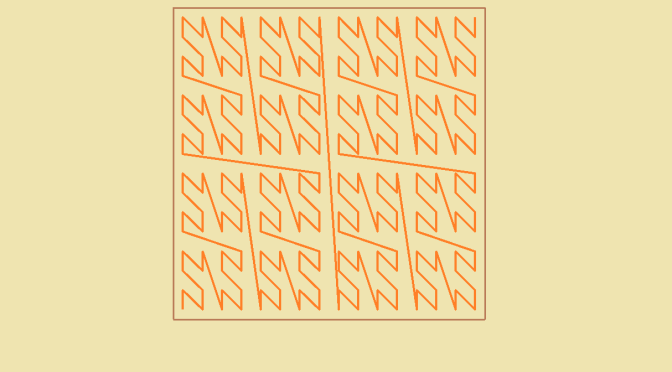# A curve filling a square – Lebesgue example

## Introduction

We aim at defining a continuous function $$\varphi : [0,1] \rightarrow [0,1]^2$$. At first sight this looks quite strange.

Indeed, $$\varphi$$ cannot be a bijection. If $$\varphi$$ would be bijective, it would also be an homeomorphism as a continuous bijective function from a compact space to a Haussdorff space is an homeomorphism. But an homeomorphism preserves connectedness and $$[0,1] \setminus \{1/2\}$$ is not connected while $$[0,1]^2 \setminus \{\varphi(1/2)\}$$ is.

Nor can $$\varphi$$ be piecewise continuously differentiable as the Lebesgue measure of $$\varphi([0,1])$$ would be equal to $$0$$.

$$\varphi$$ is defined in two steps using the Cantor space $$K$$.

## Definition of $$\varphi$$

For $$x \in K$$ there exists a unique sequence $$(a_k) \in \{0,2\}^{\mathbb{N}^*}$$ such that $$x=\sum_{k=1}^\infty a_k 3^{-k}$$. We define $$\varphi(x)=(\sum_{k=1}^\infty b_{2k} 2^{-k},\sum_{k=1}^\infty b_{2k+1} 2^{-k})$$ where $$b_k=a_k/2$$ for all $$k \in \mathbb{N}^*$$. One can verifies that $$\varphi$$ is continuous on the Cantor set relying on the fact that if two points of the Cantor set are at a distance less than $$3^{-k}$$ their ternary representations are equal for the first $$k$$ digits.

In $$x \notin K$$ then $$x$$ belongs to a unique interval whose extreme points $$r,s$$ are in $$K$$. As $$[0,1]^2$$ is convex we can define $\varphi(x)=\frac{x-r}{s-r} \varphi(s) + \frac{s-x}{s-r} \varphi(r)$ $$\varphi$$ is continuous at $$x$$. And finally, $$\varphi$$ is continuous on $$[0,1]$$.

$$\varphi$$ is onto as for $$(r=\sum_{k=1}^\infty r_k 2^{-k}, s=\sum_{k=1}^\infty s_k 2^{-k}) \in [0,1]^2$$ written with dyadic representations, we have $$\varphi(x)=(r,s)$$ for $$x=\sum_{k=1}^\infty a_k 3^{-k}$$ written with ternary representation and $$a_{2k}=2 r_k$$, $$a_{2k+1}=2 s_k$$ for all $$k \in \mathbb{N}^*$$.

One can define curves that approximate $$\varphi$$ as illustrated in above picture. This example of a curve filling a square is from Lebesgue.